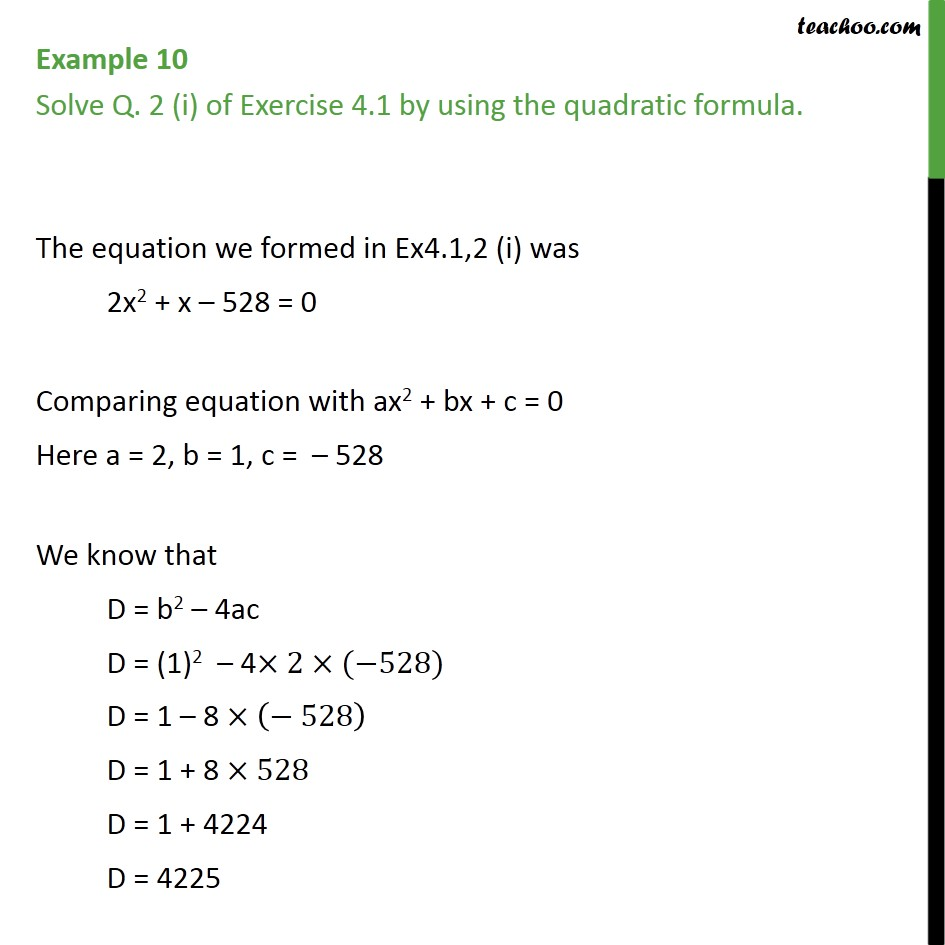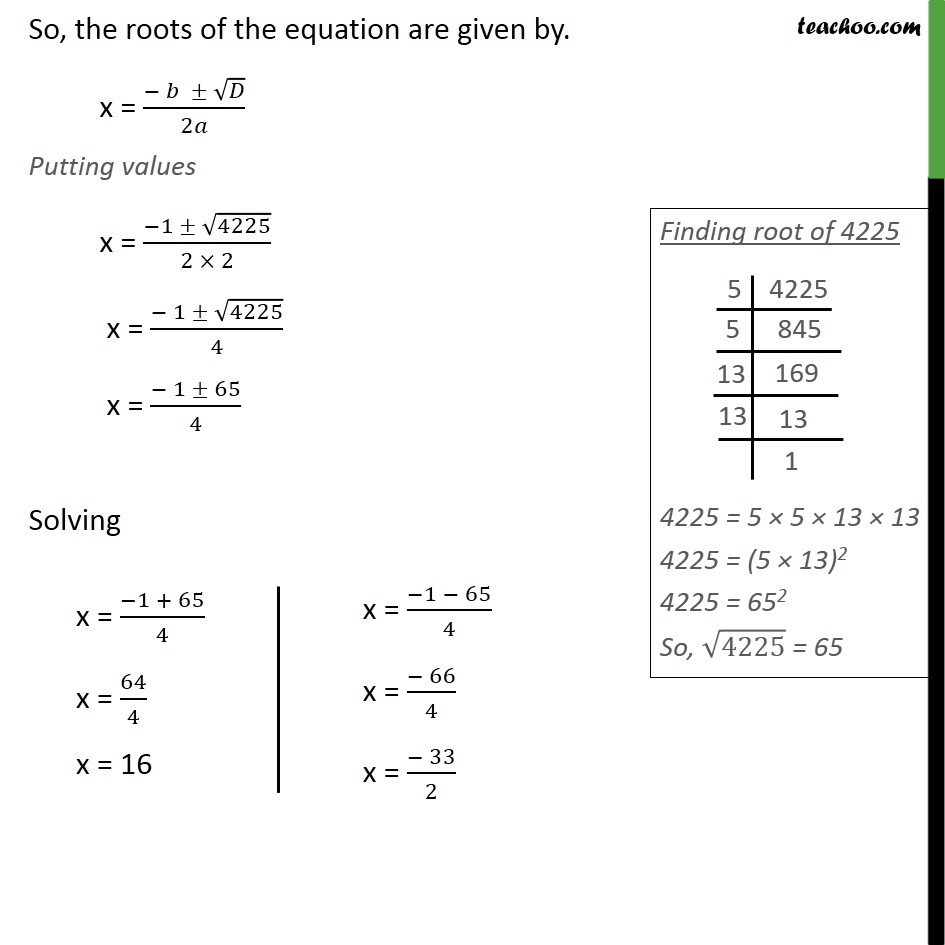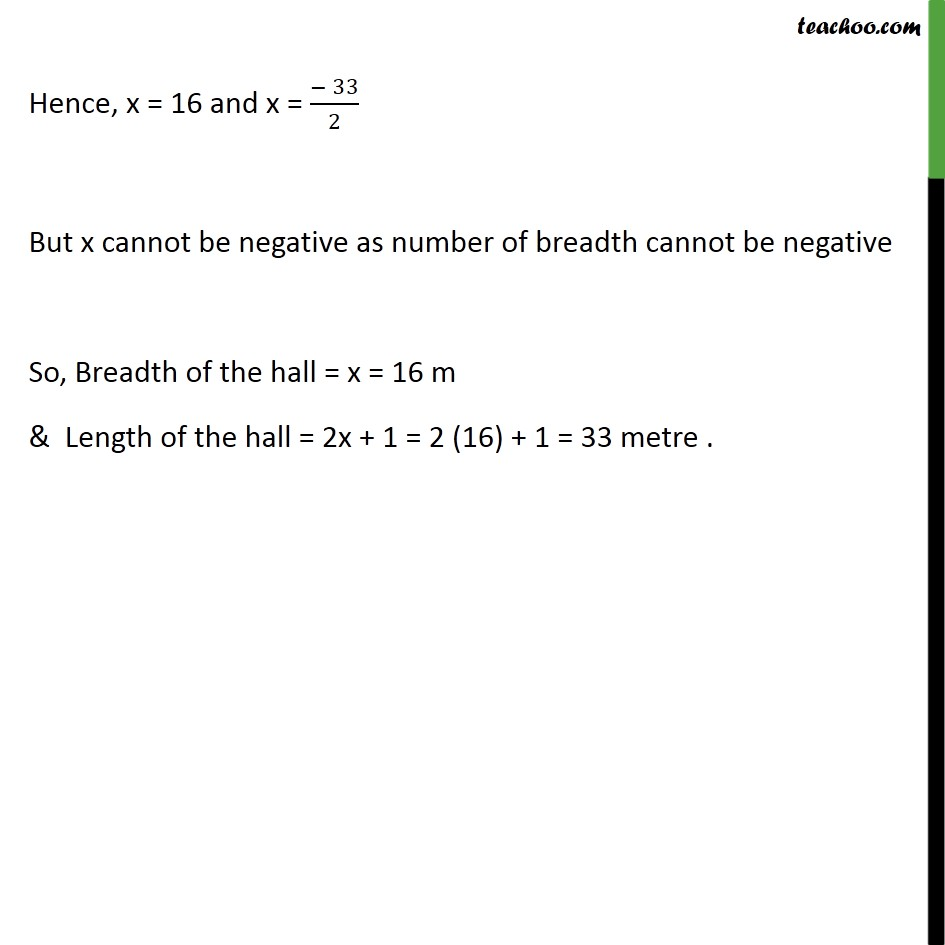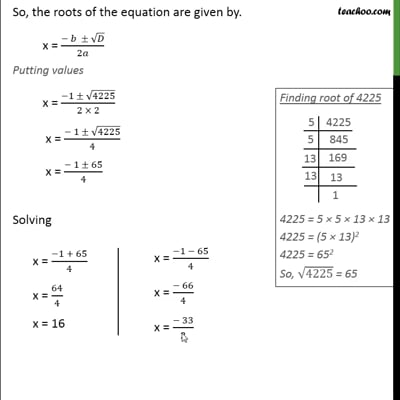Chapter 4 Class 10 Quadratic Equations

Class 10
Important Questions for Exam - Class 10This video is only available for Teachoo black users

Introducing your new favourite teacher - Teachoo Black, at only ₹83 per month

### Transcript

Example 10 Solve Q. 2 (i) of Exercise 4.1 by using the quadratic formula. The equation we formed in Ex4.1,2 (i) was 2x2 + x 528 = 0 Comparing equation with ax2 + bx + c = 0 Here a = 2, b = 1, c = 528 We know that D = b2 4ac D = (1)2 4 2 ( 528) D = 1 8 ( 528) D = 1 + 8 528 D = 1 + 4224 D = 4225 So, the roots of the equation are given by. x = ( )/2 Putting values x = ( 1 4225)/(2 2) x = ( 1 4225)/4 x = ( 1 65)/4 Solving Hence, x = 16 and x = ( 33)/2 But x cannot be negative as number of breadth cannot be negative So, Breadth of the hall = x = 16 m & Length of the hall = 2x + 1 = 2 (16) + 1 = 33 metre .